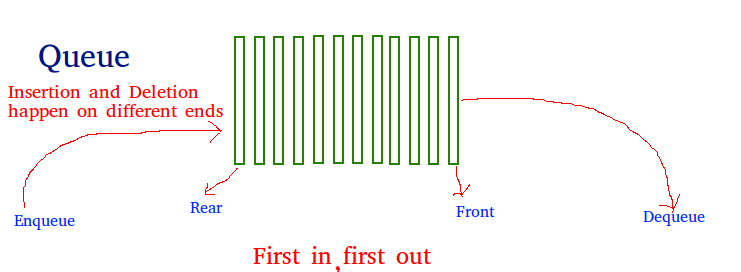# Difference between Queue and Deque in C++

• Difficulty Level : Easy
• Last Updated : 02 Nov, 2021

Queue: A Queue is a linear data structure that follows a First In First Out (FIFO) order in which the operations are performed. It is a type of container adaptor where elements are inserted into one end of the container and deleted from the other.Functions:

• empty(): Tests whether the queue is empty.
• size(): Returns the unsigned int, size of the queue.
• queue::front() and queue::back(): front() function returns a reference to the first element or the oldest of the queue. back() function returns a reference to the last or the newest element of the queue.
• push(k) and pop(): push() function adds the element ‘k’ at the end of the queue. pop() function deletes the element from the beginning of the queue and reduces its size by 1.
• swap(): exchanges the elements of two different queues of the same type but may or may not of the same size.
• emplace(): it is used to insert a new element at end of the queue.

Syntax:

`queue <data_type> q`

Below is the program to illustrate the same:

## C++

 `// C++ program to demonstrate the` `// working of queue` `#include ` `using` `namespace` `std;`   `// Driver Code` `int` `main()` `{` `    ``// Declare a queue` `    ``queue<``int``> q;`   `    ``// Insert elements in the queue` `    ``q.push(10);` `    ``q.push(5);` `    ``q.push(15);` `    ``q.push(1);`   `    ``// Delete elements from the queue` `    ``q.pop();` `    ``q.pop();`   `    ``cout << ``"Elements in Queue are: "``;`   `    ``// Print the element stored` `    ``// in queue` `    ``while` `(!q.empty()) {` `        ``cout << q.front() << ``' '``;`   `        ``// Pop the front element` `        ``q.pop();` `    ``}`   `    ``return` `0;` `}`

Output:

`Elements in Queue are: 15 1`

Deque: Deque is a sequence container with the ability of expansion and contraction on both ends. It is a template of Standard Template Library or STL in C++is. It is similar to vectors but are more efficient for the insertion and deletion of elements. Contiguous storage allocation in deque may not be guaranteed as in vectors.Functions:

• max_size(): Returns the maximum number of elements deque can contain.
• push_back() and push_front(): push_front( ) push the elements into a deque from the front and push_back( ) push elements into a deque from the back.
• pop_front() and pop_back(): pop_front() function is used to pop elements from a deque from the front and pop_back( ) function is used to pop elements from a deque from the back.
• clear() and erase():  clear is used to remove all the elements from the deque and erase is used to remove some specified elements.
• insert(): increases the container side by inserting element in the specified position.
• resize(): changes the size of the element’s container as per requirement.
• rbegin() and rend(): rbegin() points to the last element of the deque whereas rend points to the position before the beginning of the deque.
• at() and swap(): at() points to the position of the element given in the parameter and swap( ) is used two swap elements of two deques.
• emplace_front() and emplace_back(): these two functions are used to insert new elements in the container at the beginning and at the end of deque respectively.

Syntax:

`deque<data_type> dq`

Below is the program to illustrate the same:

## C++

 `// C++ program to demonstrate the` `// working of deque` `#include ` `using` `namespace` `std;`   `// Driver Code` `int` `main()` `{` `    ``// Declare a deque` `    ``deque<``int``> dq;`   `    ``// Insert element in the front` `    ``dq.push_front(10);` `    ``dq.push_front(5);` `    ``dq.push_front(3);`   `    ``// Delete elements from the the front` `    ``dq.pop_front();` `    ``dq.pop_front();`   `    ``// Insert elements in the back` `    ``dq.push_back(1);` `    ``dq.push_back(50);` `    ``dq.push_back(2);`   `    ``// Delete elements from the the back` `    ``dq.pop_back();` `    ``dq.pop_back();`   `    ``cout << ``"Elements in deque are: "``;`   `    ``// Print the element stored` `    ``// in deque` `    ``while` `(!dq.empty()) {` `        ``cout << ``" "` `<< dq.front();` `        ``dq.pop_front();` `    ``}`   `    ``return` `0;` `}`

Output:

`Elements in deque are:  10 1`

Below is the tabular difference between the queue and deque:

My Personal Notes arrow_drop_up
Recommended Articles
Page :